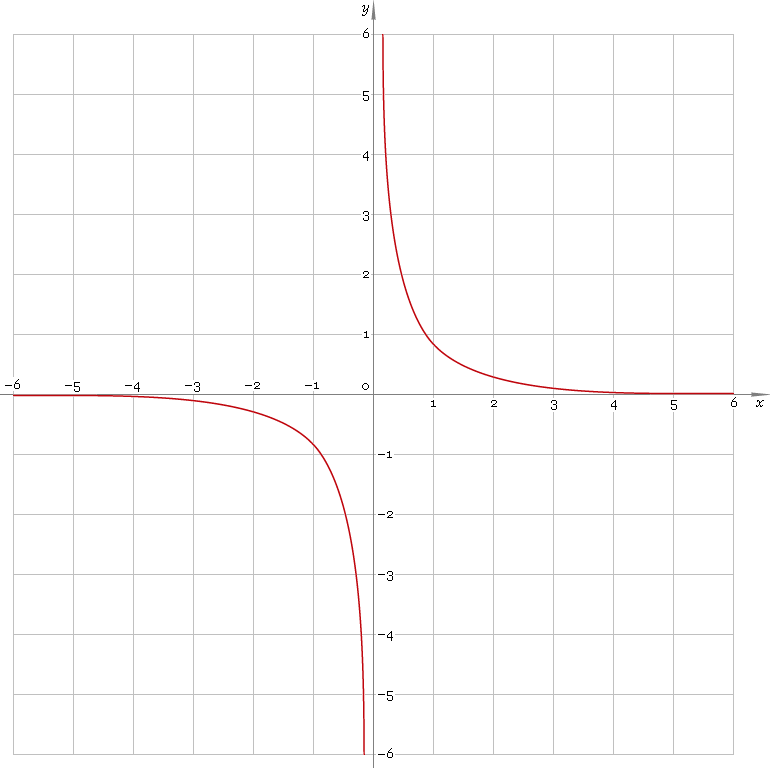The Art of Mathematics

# csch — hyperbolic cosecant function

## 1. Definition

Hyperbolic cosecant is defined as

cschx ≡ 2 /(ex − ex)

## 2. Graph

Hyperbolic cosecant is antisymmetric function defined everywhere on real axis, except its singular point 0 — so its domain is (−∞, 0)∪(0, +∞). Function graph is depicted below — fig. 1.Fig. 1. Graph of the hyperbolic cosecant function y = cschx.

Function codomain is all real axis except 0: (−∞, 0)∪(0, +∞).

## 3. Identities

Base:

coth2x − csch2x = 1

By definition:

cschx ≡ 1 /sinhx

Property of antisymmetry:

csch−x = −cschx

## 4. Derivative and indefinite integral

Hyperbolic cosecant derivative:

csch′x = −cschx cothx ≡ −coshx /sinh2x

Indefinite integral of the hyperbolic cosecant:

∫ cschx dx = ln|cothx − cschx| + C = ln|tanh(x/2)| + C

where C is an arbitrary constant.

## 5. How to use

To calculate hyperbolic cosecant of the number:

``csch(−1);``

To get hyperbolic cosecant of the complex number:

``csch(−1−i);``

To get hyperbolic cosecant of the current result:

``csch(rslt);``

To get hyperbolic cosecant of the number z in calculator memory:

``csch(mem[z]);``

## 6. Support

Hyperbolic cosecant of the real argument is supported in free version of the Librow calculator.

Hyperbolic cosecant of the complex argument is supported in professional version of the Librow calculator.